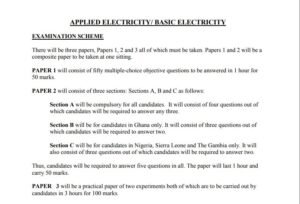WAEC News

WAEC Applied Electricity/ Basic Electricity Questions and Answers 2023 | Theory and OBJ- Download PDF. If you are about to write the West African Senior Certificate Exams (WASSCE), you will realize that you need the NECO Past Questions and Answers.

This article provides you with WAEC Applied Electricity/ Basic Electricity Questions and Answers for the WASSCE 2023. It contains information regarding the WAEC Applied Electricity/ Basic Electricity Theory and Objective Questions and Answers.Related Articles

There will be three papers, Papers 1, 2 and 3 all of which must be taken. Papers 1 and 2 will be a composite paper to be taken at one sitting.

PAPER 1 will consist of fifty multiple-choice objective questions to be answered in 1 hour for 50 marks.

PAPER 2 will consist of three sections: Sections A, B and C as follows:

Section A will be compulsory for all candidates. It will consist of four questions out of which candidates will be required to answer any three.

Section B will be for candidates in Ghana only. It will consist of three questions out of which candidates will be required to answer two.

Section C will be for candidates in Nigeria, Sierra Leone and The Gambia only. It will also consist of three questions out of which candidates will be required to answer two.

Thus, candidates will be required to answer five questions in all. The paper will last 1 hour and carry 50 marks.

PAPER 3 will be a practical paper of two experiments both of which are to be carried out by candidates in 3 hours for 100 marks.

## WAEC Applied Electricity/ Basic Electricity Objective Questions

PAPER 1
(OBJECTIVE)

1. Which of the following is a consequence of earth fault?
A. Blown fuse
B. Disconnected terminal not touching a metallic part of the appliance
C. A cut along conductor path
D. A conductor touching a metallic part of the appliance

WAEC Sample Questions and Schemes – Uploaded online by www.myschoolgist.com.ng
Use the scenario below to answer Questions 2 to 4.
In Mrs Obi’s kitchen, there are electric kettle, food blender, water heater and boiling ring. One
morning, Mrs Obi wanted to blend some fruits but the food blender could not come on. A check
at the socket outlet showed that a power source of 230 volts was available. Mrs Obi then took the
blender for repair.

2. The appliances in Mrs. Obi’s kitchen can be classified as
A. electrical appliances.
B. mechanical appliances.
C. energy appliances.
D. hand-held appliances.

3. In the food blender, the energy conversion is from
A. electrical energy to heat energy.
B. electrical energy to mechanical energy.
C. heat energy to mechanical energy.
D. electrical energy to sound energy.

4. The possible fault of the blender is
A. open circuit.
B. short circuit.
C. earth circuit.

5. The resistance of a soldering iron drawing a current of 2 A from a 230 V source is
A. 115 Ω.
B. 228 Ω.
C. 232 Ω.
D. 460 Ω.

## WAEC Applied Electricity/ Basic Electricity Theory Questions

PAPER 2
(ESSAY)

Question 1
(a) A given circuit has three resistors connected in series namely 1 Ω, 3 Ω and 4 Ω powered
by a 24 V source. Calculate the
(i) total resistance;
(ii) total current flowing through the circuit;
(iii) voltage drop across each resistor.
(b) A 15 Ω resistor and a capacitor of 20 Ω capacitive reactance are placed in parallel across a 120 V
source.
(i) Draw the circuit diagram.
(ii) Calculate the: I. current flowing in the resistor;
II. current flowing in the circuit;
III. total impedance.

Question 2
(a) Draw a 3-input circuit arrangement using switches for an:(i) OR logic gate;
(ii) AND logic gate.
(b) Using 2-input NAND, AND and OR logic gates only, draw a logic diagram
for the Boolean equation: . B + AC = Y
(c) Draw a truth table for the Boolean expression: B.C + A = Z

## WAEC Applied Electricity/ Basic Electricity Practical Questions

PAPER 3
PRACTICAL

You are provided with the following apparatus:

one d.c. power supply (0 -12 V); one d.c. milliammeter (0 – 100 mA);three 10 Ω, 1 W resistors (R1, R2 and R3)

one soldering iron with resin-cored solder;three 33 Ω, 1 W resistors (R4, R5 and R6) one toggle switch;

veroboard/ quick test board; connecting wires;

long nose pliers; side cutter.

AIM: To determine the equivalent resistance of a circuit.• Connect the circuit as shown
• Ask the supervisor to check the circuit

Table 1

• Close switch
• Adjust the power supply to obtain a reading of 3 V.
• Repeat steps (e) and (f) for the other voltages in Table
• Plot a graph of voltage V (V) on the y-axis against current I (A) on the x-axis.
• Determine the slope (L) of the
• State the equivalent resistance of the circuit.

Then call 07063986527 and drop and WhatsApp message.

Disclaimer: Please note that we are not in any way affiliated to any of the organization’s mention here. Some of the organizations here do not release their past questions, so we can only compile likely questions and answers very related to the organization.